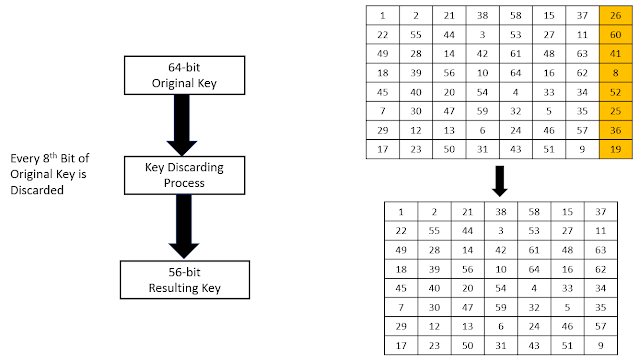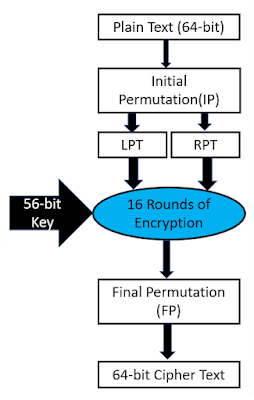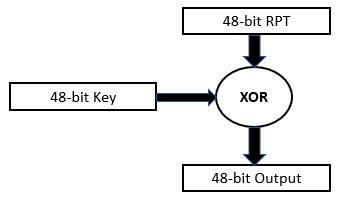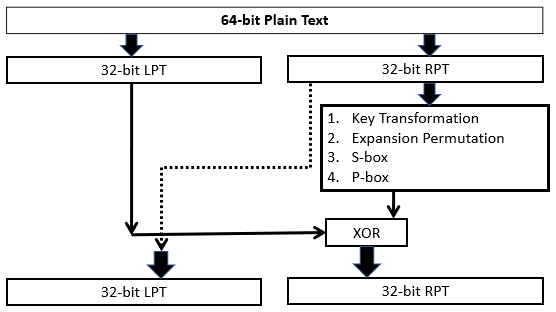## Friday, September 10, 2021

### DES Algorithm | Working of DES Algorithm | DES Encryption Process | Data Encryption Standard

DES Algorithm

Introduction

Developed in early 1970’s at IBM and submitted to NBS. DES is landmark in cryptographic algorithms. DES works based on Feistel Cipher Structure. DES is symmetric cipher algorithm and use block cipher method for encryption and decryption.Figure : Process of DESFigure : Key Discarding Process

Steps of DES

Step – 1: 64-bit plain text block is given to Initial Permutation (IP) function.

Step – 2: IP performed on 64-bit plain text block.

Step – 3: IP produced two halves of the permuted block known as Left Plain Text (LPT) and Right Plain Text (RPT).

Step – 4: Each LPT and RPT performed 16-rounds of encryption process.

Step – 5: LPT and RPT rejoined and Final Permutation (FP) is performed on combined block.

Step – 6: 64-bit Cipher text block is generated.Figure : Steps of DES

Initial Permutation (IP) & Generate LPT -RPT

Initial Permutation performed only once. Bit sequence have changed as per IP table.

For Example: 1st bit takes 40th Position, 58th bit take 1st positionOutput of IP is divided into two equal halves known as LPT, RPT. (LPT – 32 bits, RPT – 32 bit)

16 Rounds of Encryption

Step – 1: Key Transformation (56-bit key)

Key Bit Shifted per round

Compression Permutation

Step – 2: Expansion permutation of Plain Text and X-OR (P.T. size: 48 bit, C.T. size: 48 bit)

Step – 3: S-box Substitution

Step – 4: P-box (Permutation)

Step – 5: X-OR and Swap.

Figure : 16 rounds of encryption

Step – 1: Key Bit Shifted per Round

56-bit key is divided into two halves each of 28-bits.

Circular left shift is performed on each half.

Shifting of Bit position is depending on round.

For round number 1,2,9 and 16 shifts are done by one position.

For remaining rounds shift is done by 2 positions.

Compression Permutation

56-bit input with bit shifting position

Generates 48-bit key (Compression of Key bit)

Drop 9, 18, 22, 25, 35, 38, 43 and 54 bits.

Generated 48 bits keys are as below:

Step – 2: Expansion Permutation and X-OR

32-bit RPT of IP is expanded to 48-bits

Expansion permutation steps:

32-bit RPT is divided into 8-blocks each of 4-bits

48-bit RPT is XORed with 48-bit Key and output is given to S-Box.Step – 3: S-BOX Substitution

Step -4: P-BOX Permutation

Output of s-box is given to p-box

32-bit is permuted with 16 x 2 permutation table

For Example: 16th bit of S-box takes 1st Position as per below permutation table.

Step – 5: XOR and SWAP

32-bit LPT is XORed with 32-bit p-box.1st round of encryption is completed. Now remaining 15 rounds will be performed same as 1st round.

Final Permutation

At the end of the 16 rounds, the final permutation is performed (only once).

For Example: 40th bit of input takes 1st Position as per below permutation table.

The output of the final permutation is the 64-bit encrypted block (64-bit cipher text block).

Strength of DES

Key Length (Use of 56-bit Key): 256 Possible Keys (7.2 x 1016 Keys), Brute force attack takes more than thousand Years

Use of S-boxes: Complex Structure of S-box, Scope of attack is very less

DES is Reversible algorithm

Weakness of DES

Trying all 256 possible keys are not much harder these days. If you spend at least \$25 K you can build DES password crackers that will successes in few hours.

Two chosen input to an S-box can create the same output.

The purpose of initial and final permutation is not clear.

Avalanche Effect in DES

The small change in Plain text or Key produce a significant change in the Cipher text.

DES Provide a strong Avalanche effect due to complexity of algorithm.